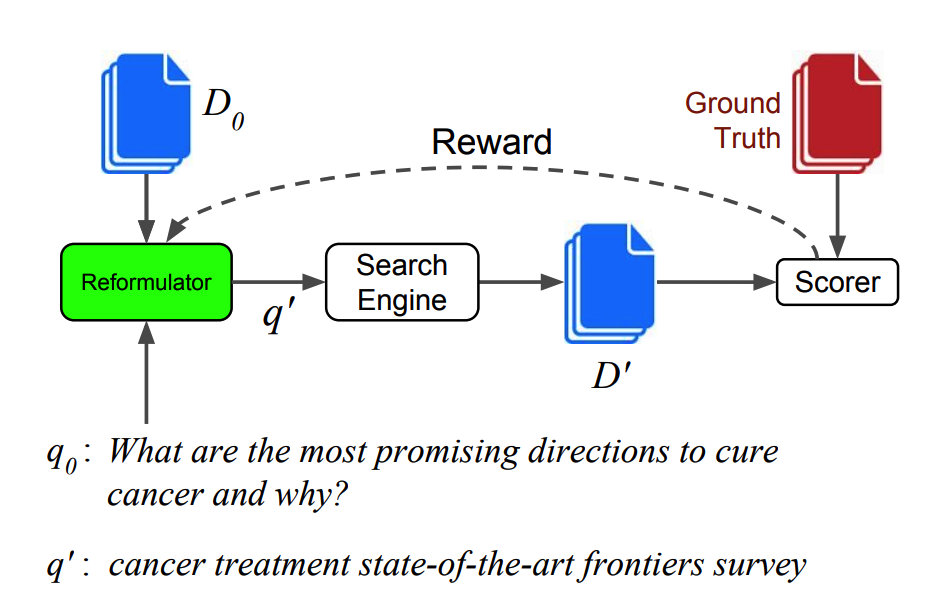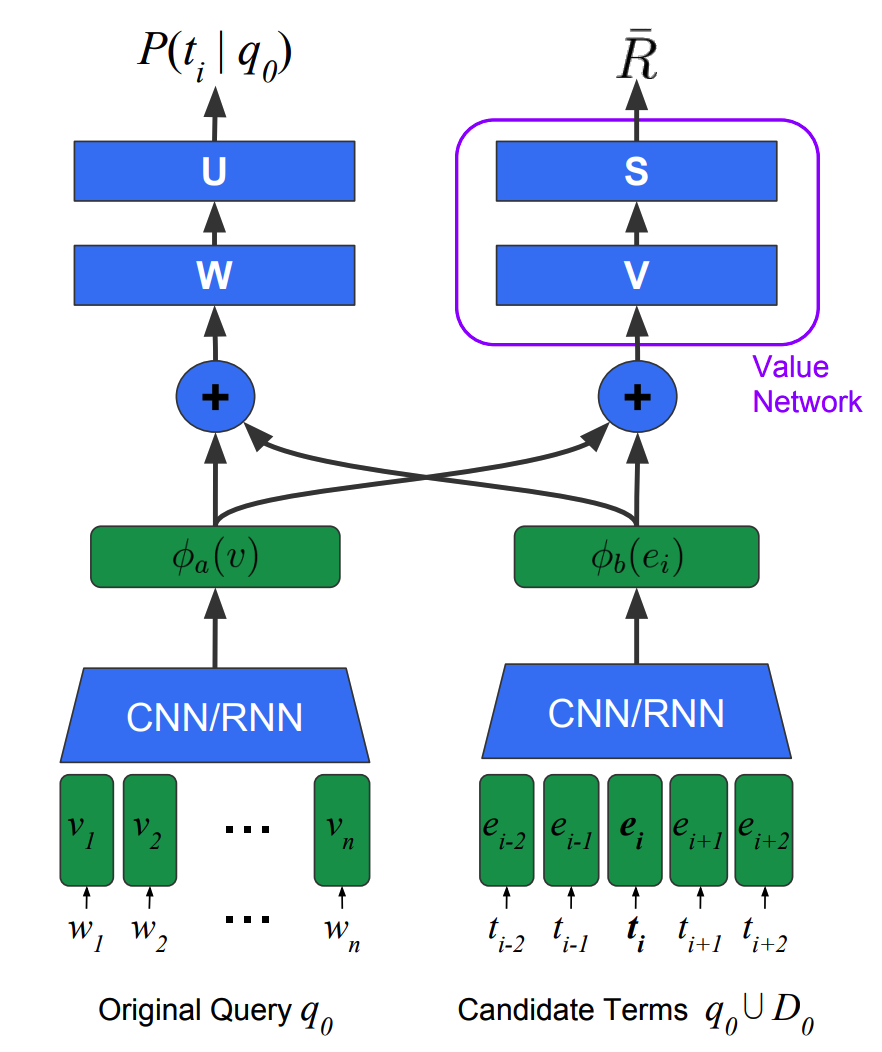# Task-Oriented Query Reformulation with Reinforcement Learning

## WHY?

Information retrieval from search engine becomes difficult when the query is incomplete or too complex. This paper suggests a query reformulation system that rewrite the query to maximize the probability of relevant documents returned.

## WHAT?Since the output of query reformulator is discrete, REINFORCE algorithm is used to train the model.The query and the candidate term vectors are converted to a fixed-sized vector using CNN with max pooling or RNN. To generate a reformulated query sequentially, LSTM is used.

\phi_a(v)\\
\phi_b(e_i)\\
e_i \in q_0 \cup D_0\\
P(t_i|q_0) = \sigma(U^{\top}tanh(W(\phi_a(v)\|\phi_b(e_i))+b))\\
P(t_i^k|q_0) \propto exp(\phi_b(e_i)^{\top}h_k\\
h_k = tanh(W_a\phi_a(v)+W_b\phi_b(t^{k-1}+W_h h_{k-1})

To train the model, REINFORCE algorithm is used. Entropy regularization loss is add to encourage diversity.

C_a = (R - \bar{R})\sum_{t\in T} - log P(t|q_0)\\
\bar{R} = \sigma(S^{\top}tanh(V(\phi_a(v)\|\bar{e})+b))\\
\bar{e} = \frac{1}{N}\sum_{i=1}^N \phi_b(e_i)\\
N = |q_0 \cup D_0|\\
C_b = \alpha\|R - \bar{R}\|^2\\
C_H = -\lambda \sum_{t\in q_0\cup D_0} P(t|q_0) log P(t|q_0)

## So?

Query reformulation showed better performance than raw retrieval, PRF models, or SL methods in TREC-CAR, Jeopardy and MSA data measured in recall, precision and mean average precision.

Powered by aiden